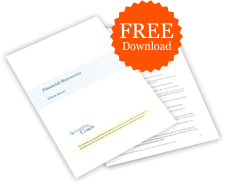# What is the effective interest rate for a bond?

## Definition of Effective Interest Rate of a Bond

The effective interest rate of a bond is usually the market interest rate and the bond's yield-to-maturity (as opposed to the interest rated stated on the face of the bond).

The effective interest rate of a bond is the rate that will discount both the bond's future interest payments and the bond's maturity value to a present value that is equal to the bond's current market value. If the market interest rate increases, the present value (and the market value) of the bond will decrease. If the market interest rate decreases, the present value (and the market value) of the bond will increase.

## Example of Effective Interest Rate of a Bond

Assume that an investor wants to purchase an existing bond. One bond that is available has a face/maturity value of \$100,000 and has a stated interest rate of 5% and will mature in 3 years. The cash that the investor will be receiving is \$2,500 every six months (\$100,000 X 5% X 1/2 year) for 3 years and then \$100,000 at the end of 3 years. The bond's current price is \$83,748. At this price, the investor will earn an effective interest rate (or a yield to maturity) of 6% as shown here:

The present value of the cash from a 5% \$100,000 bond maturing in 3 years and trading in a 6% market is as follows:

• \$2,500 every six months for 3 years discounted by 3% semiannually = \$13,543
• \$100,000 at the end of 3 years discounted by 3% semiannually = \$83,748
• Total present value, total market value = \$97,291### Free Financial Statements Cheat Sheet

543,611
Subscribers
You are already subscribed. This offer is not available to existing subscribers.
Error: You have unsubscribed from this list.